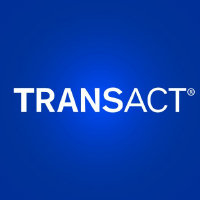# TransAct Technologies Inc NASDAQ:TACTTransAct Technologies Inc
NASDAQ:TACT
Price: 7.07 USD +1.29%
Updated:

## DCF Value

This DCF valuation model was created byAlpha Spread and was last updated on Nov 25, 2023.

Estimated DCF Value of oneTACT stock is 1.96 USD. Compared to the current market price of 7.07 USD, the stock is Overvalued by 72%.

DCF Valuation FAQ:
TACT DCF Value
Base Case
1.96 USD
Overvaluation 72%
DCF Value
Price1.96
DCF Value
TransAct Technologies Inc Competitors:
DCF Valuation

Revenue
62.8m 86.2m
Net Income
2.9m 2.3m

## Present Value Calculation

The first step in the DCF calculation process is to estimate the company's future free cash flow. Once free cash flow is forecasted, it is discounted at a risk-appropriate discount rate. The resulting value is the present value of the company's free cash flow. You can change any model inputs below.

Available Valuation Models
1 valuation model availableUpdated on Nov 25, 2023
Default Valuation
Other investors haven't shared any valuation models for TransAct Technologies Inc.
Model Settings
Operating Model
Discount Rate
8.8%
Forecast Period
5 Years
Terminal Growth
0%
Discount Rate
8.8%
Terminal Growth
0%
Growth Period
5 Years

### DCF Model Base Case ScenarioDCF Model
Currency: USD
Thousands

To view the process of deriving the DCF Value of one share from the estimated Present Value, see the DCF Value Calculation block.

## DCF Value Calculation

Depending on which type of operating model is chosen (equity or whole firm valuation model), the resulting value is either the value of equity or the value of the entire firm. In the case of the latter, to move from the value of the firm to the value of equity, liabilities are subtracted and assets are added.

### Capital Structure From Present Value to DCF ValuePresent Value 19.5m USD Equity Value 19.5m USD / Shares Outstanding 10m TACT DCF Value 1.96 USD
Overvalued by 72%

To view the process of calculating the Present Value of TransAct Technologies Inc' future free cash flow, see the Present Value Calculation block.

Estimated DCF Value of oneTACT stock is 1.96 USD. Compared to the current market price of 7.07 USD, the stock is Overvalued by 72%.
1. Present Value Calculation. Using the DCF Operating Model we projectTransAct Technologies Inc's future free cash flow and discount it at a selected discount rate to calculate its Present Value (19.5m USD).
2. DCF Value Calculation. We use the company's capital structure to calculate the total Equity Value based on the previously computed Present Value of the free cash flow. Dividing the Equity Value by the number of shares outstanding gives us the DCF Value of 1.96 USD per oneTACT share.